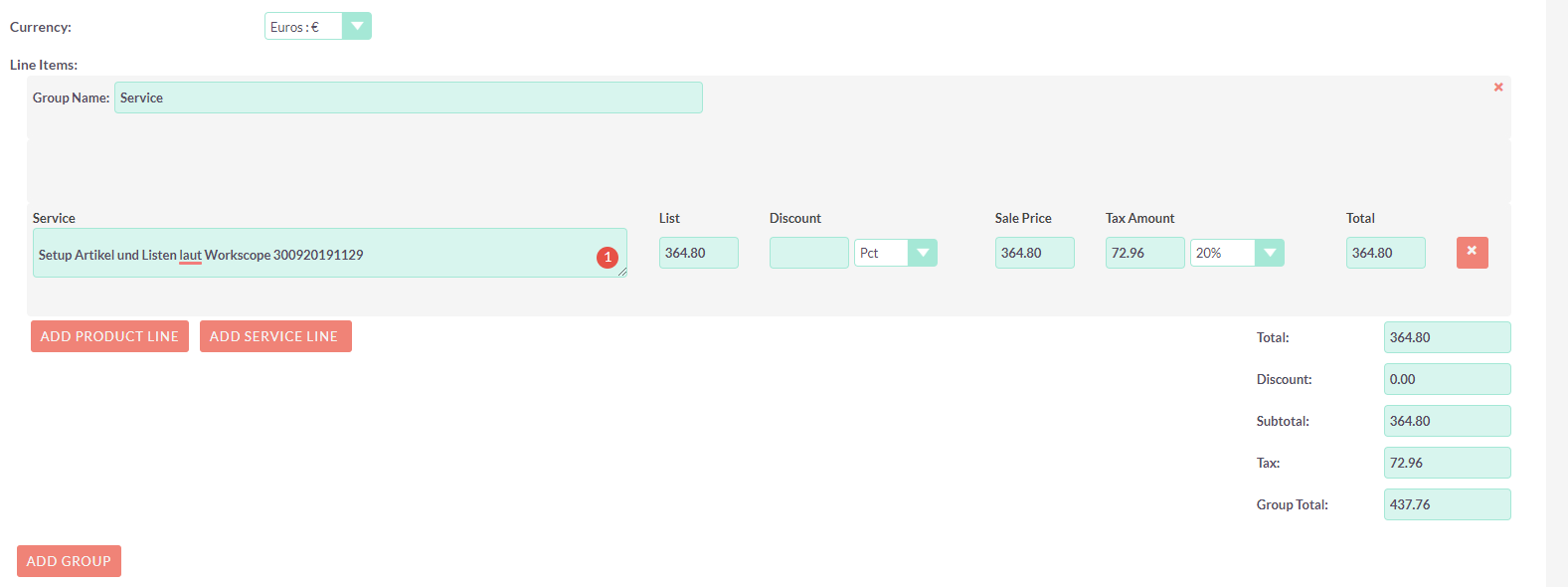# Calculation of taxes are wrong

When i set 20% tax in my quote it calculates wrong:

I communicated 456 (gross) to my customer which included 20% VAT
When I substract the 20% i get 364,80

List: 364,80
Tax Amount (set to 20%): is 76 (only 16%?)
Group Total: 437.76

Am I missing something? I have’nt changed anything in this regards, so I don’t know why its only calculatin 16% when I select 20%)

Can you share a screenshot of the Full Line Items and the values set there? Do you have anything set in Discount or Sale Price?attached

the thing in the image it shows correctly. but my original gross amount was 456 which 20% of it would be 91,20. So I substracted that amount from gross to receive net. now when i use 364,8 as net and add 20% as tax it doesnt show the 456 (original amount).

There is a misunderstanding in your calculation. You are doing 20% of 456 = 91,20 and then you think that subtracting 91,20 from 456 (= 364) would give you 456 if you do a 20% on 364. To simplify

20% of 456 and 20% of 364 would not be equal.
Your actual Net amount is 380. Please use this calculator to find out NET by providing Gross and Tax %.

geez… now that I see “380” I remember sending this amount to my client as a net amount.
yes, I definitely had a broken link right thereThanks for pointing it out!